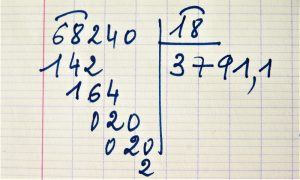Mathematics

# Rational numbers

In mathematics, numbers can be classified according to their characteristics and use. Rational numbers represent the set of numbers that can be fractionated to talk about the parts of a whole. These numbers are frequently used to represent measurements in different areas such as architecture, medicine, chemistry, biology, etc.Related topics

## What are rational numbers?

Rational or fractional numbers are those that can be described through a fraction. They are represented by the consonant Q that comes from the Italian word "Quoziente", which is translated as quotient. They are composed of integers, zero and fractional numbers.

## Which are the rational numbers?

Rational numbers are integers and fractional numbers:

• Integers are numbers that do not have decimals. Example: 3
• Zero is a null value number that represents that there is no number or element to count. In the case of rational numbers, zero can be accompanied by decimals. Example: 0,5
• Fractional numbers are non-integer numbers, e.g. 2/6, 4/5, 6/9.

## Classification

Rational numbers can be classified into:

• Not null (Q*): are all rational numbers discarding zero.
• Not negative (Q+): are all positive rational numbers and zero.
• Not positive (Q-): all negative rational numbers and zero.
• Positive (Q*+): all positive numbers except zero.
• Negative (Q*-): all negative numbers not including zero.
• Decimal numbers: are those that can be written in fractions.

Depending on their decimal expression, decimal numbers can be classified as limited rational or periodic numbers.

Limited numbers are those that have a fixed decimal representation. Example ½ = 0.5

Periodic numbers are the ones that have an unlimited number of digits. These can be pure periodicals or mixed periodicals. Pure periodical numbers have a pattern after the comma, Example: 5.333333,

Mixed periodic numbers have a pattern after the determined number. Example: 5.541472727272727272.

## What are they for?

Rational numbers are used to express measures in elements that we can fractionate. For example, if we talk about a cake that we divided in 4 pieces and we eat one of them, we can say that we ate ¼ (0,25) of the cake and if we ate the four pieces 4/4 (1) we will have eaten the whole cake.

In addition, thanks to this type of numbers, learning the operations to divide is facilitated.

## Characteristics of rational numbers

Among the most outstanding characteristics of rational numbers, the following can be mentioned:

• They are infinite.
• They can be expressed in fractions or with decimals.
• They represent one or several parts of an integer.

## History

The history of rational numbers has an unknown origin. However, in ancient times, it is the Egyptians who make greater use of these numbers to solve their problems using fractions of an integer.

In Egypt, they were used to solve problems in the construction area.

In ancient Greece, the numbers take the name because the word rational comes from the Latin ratio meaning reason or separation. At this time, Pythagorean mathematics used these numbers to express commensurable magnitudes in different disciplines such as construction, music, anatomy, etc.

In modern times, they are identified with the notation Q which is the initial of the Italian word “Quoziente” thanks to the works made by Italian mathematician Giuseppe Peano in 1895.

These numbers are widely used in mathematics teaching and all kinds of fractional operations. In the professional field, rational numbers make it possible to obtain exact measurements in the construction of pieces of metal, wood and other materials; they allow us to obtain the real weight of food or objects; they are represented in the measurement tables of medical and chemical utensils, among others.

## How are they represented?

Rational numbers are represented by the letter Q which is the first letter of the Italian word “Quoziente”, which is translated as quotient. This representation comes from the works of Giuseppe Peano in 1895 about this numerical set.

## Properties

Rational numbers are used in mathematical operations such as addition, subtraction, multiplication and division, and in each of these, the numerical set has the following properties.

• Properties of rational numbers for addition and subtraction:
• Internal property
• Associative ownership
• Commutative property
• Neutral element (the number 0)
• Reverse additive or opposite element
• Properties for multiplication and division:
• Internal property
• Associative ownership
• Commutative property
• Distributive ownership
• Neutral element (number 1)

## Operations

With rational numbers we can perform operations such as addition, subtraction, multiplication and division.

## Examples of rational numbers

Below are several examples of rational numbers.

#### Example of non-nulls (Q*)

Are all the numbers discarding zero.

½ = 0,5

#### Example of no negatives (Q+)

All the positive numbers and zero

¼= 0,25

#### Example of not positive (Q-)

All the negative numbers and zero.

-7/8

#### Example of positives (Q*+)

All the positive numbers except zero.

7/14

#### Example of negatives (Q*-)

All the negative numbers not including zero.

-2/4

#### Example of decimal numbers

Are the ones that can be written in fractions.

3,15

Written by Gabriela Briceño V.